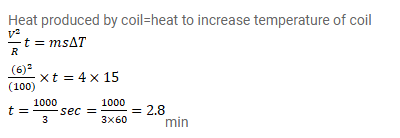# A coil of resistance

Question:

A coil of resistance $100^{\Omega}$ is connected across a battery of emf $6.0 \mathrm{~V}$. Assume that the heat developed in the coil is used to raise its temperature. If the heat capacity of the coil is $4.0 \mathrm{~J} / \mathrm{K}$, how long will it take to raise the temperature of the coil by 15 ${ }^{\circ} \mathrm{C}_{\text {? }}$

Solution: